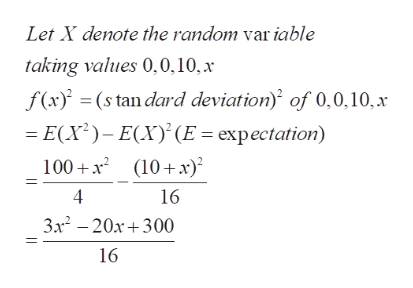# This is a standard deviation contest. You must choose four numbers from the whole numbers 0 to 10, with repeats allowed.The four numbers that have the largest possible standard deviation are 0, 0, 10 and _______.(Give your answer as a whole number.)

Question

This is a standard deviation contest. You must choose four numbers from the whole numbers 0 to 10, with repeats allowed.

The four numbers that have the largest possible standard deviation are 0, 0, 10 and _______.

check_circleExpert Solution
Step 1

To determine the number x from {0,1,2,...,10] such that the standard deviation of 0,0,10 and x is the largest

Step 2

We need to find the value of x which maximizes the expression in x  shown above,help_outlineImage TranscriptioncloseLet X denote the random var iable taking values 0,0,10, x f(x)(s tan dard deviation) of 0,0,10, x E(X)-E(X(E expectation 100x (10 x) 4 16 3x2 -20x300 16 fullscreen
Step 3

Method 1: the derivative of the numerator is 6x-20, so the function inc...

### Want to see the full answer?

See Solution

#### Want to see this answer and more?

Solutions are written by subject experts who are available 24/7. Questions are typically answered within 1 hour*

See Solution
*Response times may vary by subject and question
Tagged in

### Basic Probability